# 二十二、学习识别交通标志

• 监督学习概念
• 用于特征提取的德国交通标志识别基准GTSRB）数据集
• 支持向量机SVM

1. 预处理数据集：我们需要一种加载数据集，提取兴趣区域并将数据拆分为适当的训练和测试集的方法。
2. 提取特征：可能是原始像素值不是数据的最有信息的表示。 我们需要一种从数据中提取有意义的特征的方法，例如基于不同颜色空间和 HOG 的特征。
3. 训练分类器：我们将以两种不同的方式在训练数据上训练多分类器：一对多策略（我们为每个类别训练单个 SVM，该类别的样本为正面样本，所有其他样本为负例），以及与一对策略（我们为每对类别训练一个 SVM） ，其中第一类样本为正面样本，第二类样本为负面样本）。
4. 为分类器评分：我们将通过计算不同的表现指标（例如准确率，准确率和召回率）来评估经过训练的集成分类器的质量。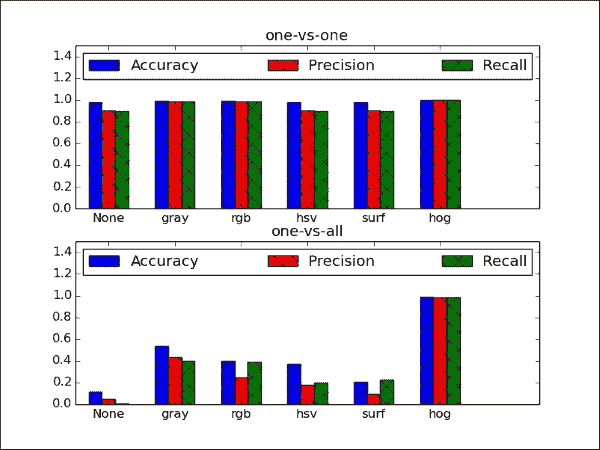# 规划应用

• main：用于启动应用的main函数例程（在chapter6.py中）。
• datasets.gtsrb：用于解析德国交通标志识别基准（GTSRB）数据集的脚本。 该脚本包含以下函数：
• load_data：用于加载 GTSRB 数据集，提取所选特征并将数据分为训练集和测试集的函数。
• _extract_featuresload_data调用此函数以从数据集中提取所选特征。
• classifiers.Classifier：一个抽象基类，为所有分类程序定义公共接口。
• classifiers.MultiClassSVM：使用以下公共方法为多类分类实现 SVM 集成的类：
• MultiClassSVM.fit：一种用于将 SVM 的集合适合训练数据的方法。 它以训练数据矩阵作为输入，其中每一行是训练样本，列中包含特征值和标签向量。
• MultiClassSVM.evaluate：一种用于通过在训练后将 SVM 应用于某些测试数据来评估 SVM 整体的方法。 它以测试数据矩阵作为输入，其中每一行都是测试样本，各列包含特征值和标签向量。 该函数返回三种不同的表现指标：准确率，准确率和召回率。

# 监督学习

## 训练程序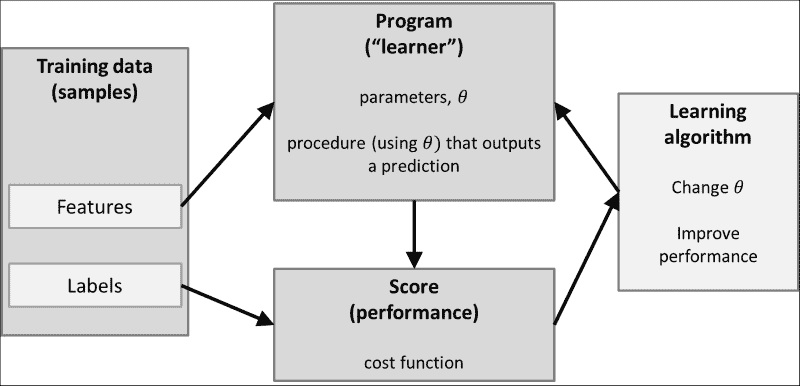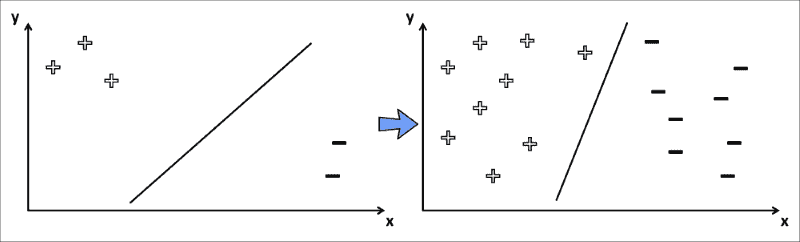## 测试程序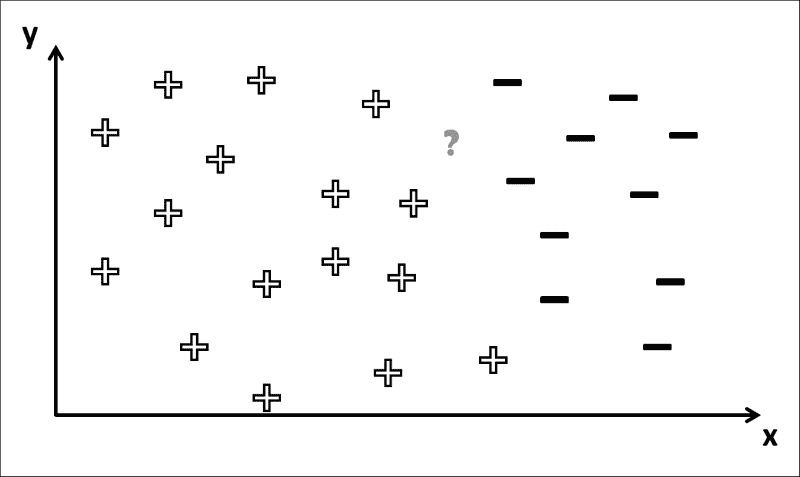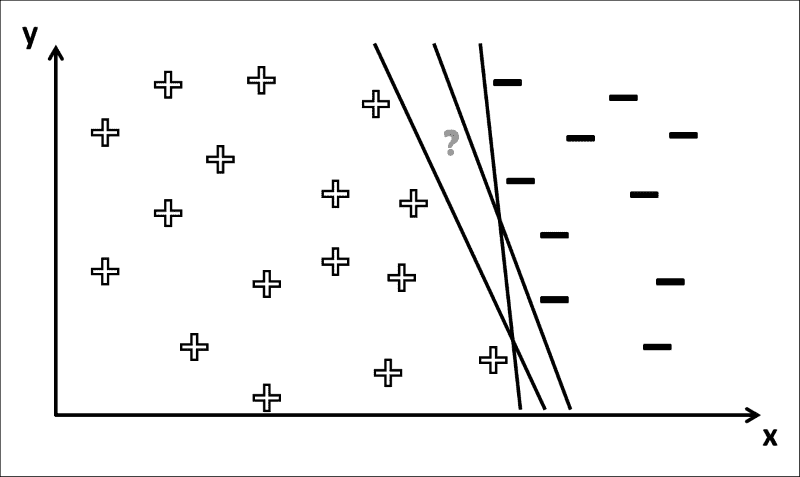## 分类器基类

from abc import ABCMeta


class Classifier:
"""Abstract base class for all classifiers"""
__metaclass__ = ABCMeta


    @abstractmethod
def fit(self, X_train, y_train):
pass

@abstractmethod
def evaluate(self, X_test, y_test, visualize=False):
pass


# GTSRB 数据集

GTSRB 数据集非常大，有条理，开源且带有注释，因此非常适合我们的目的。 但是，出于本书的目的，我们将分类限制为总共 10 个类别的数据样本。

sample_size = 15
sample_idx = np.random.randint(len(X), size=sample_size)
sp = 1
for r in xrange(3):
for c in xrange(5):
ax = plt.subplot(3,5,sp)
sample = X[sample_idx[sp-1]]
ax.imshow(sample.reshape((32,32)), cmap=cm.Greys_r)
ax.axis('off')
sp += 1
plt.show()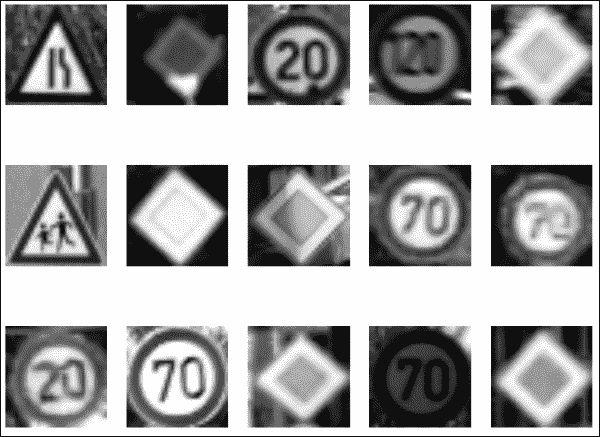## 解析数据集

import cv2
import numpy as np
import csv

from matplotlib import cm
from matplotlib import pyplot as plt

test_split=0.2, plot_samples=False, seed=113):


classes = np.array([0, 4, 8, 12, 16, 20, 24, 28, 32, 36])


X = [] # images
labels =  []  # corresponding labels

# subdirectory for class
for c in xrange(len(classes)):
prefix = rootpath + '/' + format(classes[c], '05d') + '/'

# annotations file
gt_file = open(prefix + 'GT-'+ format(classes[c], '05d')+ '.csv')


    gt_reader.next() # skip header
# loop over all images in current annotations file
# first column is filename


    if cut_roi:
im = im[np.int(row):np.int(row),
np.int(row):np.int(row), :]


    X.append(im)
labels.append(c)
gt_file.close()


if feature is not None:
X = _extract_feature(X, feature)


np.random.seed(seed)
np.random.shuffle(X)
np.random.seed(seed)
np.random.shuffle(labels)

X_train = X[:int(len(X)*(1-test_split))]
y_train = labels[:int(len(X)*(1-test_split))]
X_test = X[int(len(X)*(1-test_split)):]
y_test = labels[int(len(X)*(1-test_split)):]


return (X_train, y_train), (X_test, y_test)


# 特征提取

• 一些简单的颜色转换，例如灰度，RGB 和 HSV。 基于灰度图像的分类将为分类器提供一些基线表现。 由于某些交通标志的颜色方案不同，RGB 可能会给我们带来更好的表现。 HSV 有望提供更好的表现。 这是因为它代表的颜色甚至比 RGB 更坚固。 交通标志往往具有非常明亮，饱和的颜色（理想情况下）与周围环境完全不同。
• 加速鲁棒特征SURF），现在应该对您来说非常熟悉。 我们以前已经认识到 SURF 是一种从图像中提取有意义的特征的有效且鲁棒的方法，因此我们不能在分类任务中利用此技术来获得优势吗？
• 定向梯度直方图HOG），它是迄今为止本章要考虑的最高级特征描述符。 该技术可以计算沿图像上排列的密集网格出现的梯度方向，非常适合与 SVM 一起使用。

def _extract_feature(X, feature):
# operate on smaller image
small_size = (32, 32)
X = [cv2.resize(x, small_size) for x in X]


## 通用预处理

# normalize all intensities to be between 0 and 1
X = np.array(X).astype(np.float32) / 255

# subtract mean
X = [x - np.mean(x) for x in X]


## 灰度特征

if feature == 'gray' or feature == 'surf':
X = [cv2.cvtColor(x, cv2.COLOR_BGR2GRAY) for x in X]


## 色彩空间

if feature == 'hsv':
X = [cv2.cvtColor(x, cv2.COLOR_BGR2HSV) for x in X]


## 加速鲁棒

if feature == 'surf':
# create dense grid of keypoints
dense = cv2.FeatureDetector_create("Dense")
kp = dense.detect(np.zeros(small_size).astype(np.uint8))


surf = cv2.SURF(400)
surf.upright = True
surf.extended = True


kp_des = [surf.compute(x, kp) for x in X]


num_surf_features = 36
X = [d[:num_surf_features, :] for d in kp_des]


## 定向梯度直方图

HOG 特征背后的基本思想是图像中对象的局部形状和外观可以通过边缘方向的分布来描述。 图像被划分为较小的连接区域，在该区域中会编译梯度方向（或边缘方向）的直方图。 然后，通过连接不同的直方图来组装描述符。 为了提高表现，可以对局部直方图进行对比度归一化，从而更好地保持照明和阴影变化的不变性。 您会看到为什么这种预处理可能恰好适合于在不同视角和光照条件下识别交通标志。

HOG 描述符可以通过cv2.HOGDescriptor在 OpenCV 中完全访问，它采用检测窗口大小（32 x 32），块大小（16 x 16），像元大小（8 x 8）和像元跨度（8 x 8），作为输入参数。 然后，对于这些单元格中的每个单元格，HOG 描述符使用九个面元计算定向梯度的直方图：

elif feature == 'hog':
block_size = (small_size / 2, small_size / 2)
block_stride = (small_size / 4, small_size / 4)
cell_size = block_stride
num_bins = 9
hog = cv2.HOGDescriptor(small_size, block_size, block_stride, cell_size, num_bins)


X = [hog.compute(x) for x in X]


X = [x.flatten() for x in X]
return X


# 支持向量机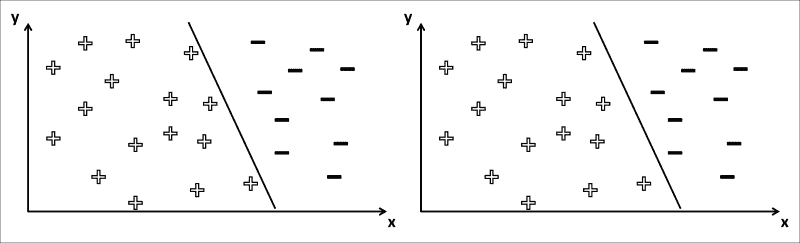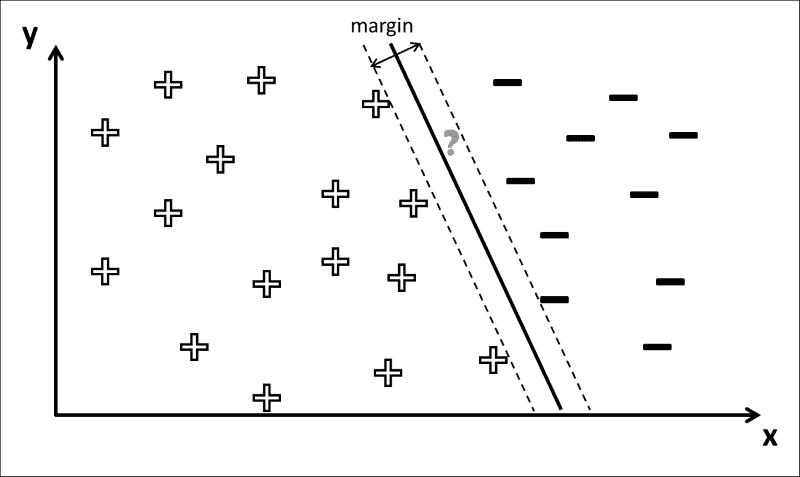## 使用 SVM 的多类别分类

• 一对多：一对多策略涉及每个类别训练一个分类器，该类别的样本为正面样本，所有其他样本为负例。 因此，对于k类，此策略需要训练k不同的 SVM。 在测试期间，所有分类器都可以通过预测未见样本属于其类别来表示“+1”投票。 最后，合奏将看不见的样本分类为投票最多的类别。 通常，此策略与置信度分数结合使用，而不是与预测标签结合使用，以便最终可以选择置信度分数最高的类。
• 一对一：一对一策略涉及每类对训练一个分类器，第一类样本为正面样本，第二类样本为负面样本。 对于k类，此策略需要训练k*(k-1)/2分类器。 但是，分类器必须解决一个非常简单的任务，因此在考虑使用哪种策略时需要权衡取舍。 在测试过程中，所有分类器都可以对第一或第二个类别表示+1投票。 最后，合奏将看不见的样本分类为投票最多的类别。

class MultiClassSVM(Classifier):
"""Multi-class classification using Support Vector Machines (SVMs) """
def __init__(self, num_classes, mode="one-vs-all", params=None):
self.num_classes = num_classes
self.mode = mode
self.params = params or dict()


        # initialize correct number of classifiers
self.classifiers = []
if mode == "one-vs-one":
# k classes: need k*(k-1)/2 classifiers
for i in xrange(numClasses*(numClasses-1)/2):
self.classifiers.append(cv2.SVM())
elif mode == "one-vs-all":
# k classes: need k classifiers
for i in xrange(numClasses):
self.classifiers.append(cv2.SVM())
else:
print "Unknown mode ",mode


## 训练 SVM

def fit(self, X_train, y_train, params=None):
""" fit model to data """
if params is None:
params = self.params


if self.mode == "one-vs-one":
svm_id=0
for c1 in xrange(self.numClasses):
for c2 in xrange(c1+1,self.numClasses):


y_train_c1 = np.where(y_train==c1)
y_train_c2 = np.where(y_train==c2)

data_id = np.sort(np.concatenate((y_train_c1, y_train_c2), axis=0))
X_train_id = X_train[data_id,:]
y_train_id = y_train[data_id]


y_train_bin = np.where(y_train_id==c1, 1, 0).flatten()


self.classifiers[svm_id].train(X_train_id, y_train_bin, params=self.params)


svm_id += 1


elif self.mode == "one-vs-all":
for c in xrange(self.numClasses):


y_train_bin = np.where(y_train==c,1,0).flatten()
self.classifiers[c].train(X_train, y_train_bin, params=self.params)


OpenCV 将负责其余的工作。 实际情况是，SVM 训练使用拉格朗日乘数来优化一些约束，这些约束会导致最大利润的决策边界。 通常会执行优化过程，直到满足某些终止条件为止，这些条件可以通过 SVM 的可选参数指定：

params.term_crit = (cv2.TERM_CRITERIA_EPS + cv2.TERM_CRITERIA_MAX_ITER, 100, 1e-6)


## 测试 SVM

def evaluate(self, X_test, y_test, visualize=False):
"""Evaluates model performance"""
Y_vote = np.zeros((len(y_test), self.numClasses))


if self.mode == "one-vs-one":
svm_id = 0
for c1 in xrange(self.numClasses):
for c2 in xrange(c1+  1, self.numClasses):
data_id = np.where((y_test==c1) + (y_test==c2))
X_test_id = X_test[data_id,:],:],:]
y_test_id = y_test[data_id]

# predict labels
y_hat = self.classifiers[svm_id].predict_all( X_test_id)


            # we vote for c1 where y_hat is 1, and for c2 where
# y_hat is 0
# np.where serves as the inner index into the
# data_id array, which in turn serves as index
# into the Y_vote matrix
Y_vote[data_id[np.where(y_hat==1)],c1] += 1


            Y_vote[data_id[np.where(y_hat==0)],c2] += 1


             svm_id += 1


“一对多”策略提出了一个不同的问题。 在Y_vote矩阵中建立索引的难度较小，因为我们始终考虑所有测试数据样本。 我们为每个数据样本重复预测标签的过程：

elif self.mode == "one-vs-all":
for c in xrange(self.numClasses):
# predict labels
y_hat = self.classifiers[c].predict_all(X_test)


        # we vote for c where y_hat is 1
if np.any(y_hat):
Y_vote[np.where(y_hat==1), c] += 1


        # find all rows without votes, pick a class at random
no_label = np.where(np.sum(y_vote,axis=1)==0)
Y_vote[no_label,np.random.randint(self.numClasses, size=len(no_label))] = 1


accuracy = self.__accuracy(y_test, Y_vote)
precision = self.__precision(y_test, Y_vote)
recall = self.__recall(y_test, Y_vote)

return (accuracy,precision,recall)


### 注意

scikit-learn机器学习包支持即开即用的三个指标：准确率，准确率和召回率（以及其他） ，还附带了各种其他有用的工具。 出于教育目的（并最大程度地减少软件依赖项），我们将自己得出这三个指标。

### 混淆矩阵

def __confusion(self, y_test, Y_vote):
y_hat = np.argmax(Y_vote, axis=1)


    conf = np.zeros((self.numClasses, self.numClasses)).astype(np.int32)
for c_true in xrange(self.numClasses):
# looking at all samples of a given class, c_true
# how many were classified as c_true? how many as others?
for c_pred in xrange(self.numClasses):


            y_this = np.where((y_test==c_true) * (y_hat==c_pred))


            conf[c_pred,c_true] = np.count_nonzero(y_this)


    return conf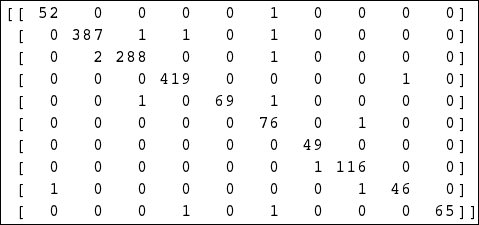### 准确率

def __accuracy(self, y_test, y_vote):
""" Calculates the accuracy based on a vector of ground-truth labels (y_test) and a 2D voting matrix (y_vote) of size (len(y_test),numClasses). """


    y_hat = np.argmax(y_vote, axis=1)


    # all cases where predicted class was correct


    return np.count_nonzero(mask)*1./len(y_test)


### 精度

def __precision(self, y_test, Y_vote):
""" precision extended to multi-class classification """
# predicted classes
y_hat = np.argmax(Y_vote, axis=1)


    if True or self.mode == "one-vs-one":
# need confusion matrix
conf = self.__confusion(y_test, y_vote)

# consider each class separately
prec = np.zeros(self.numClasses)
for c in xrange(self.numClasses):


            # true positives: label is c, classifier predicted c
tp = conf[c,c]


            # false positives: label is c, classifier predicted
# not c
fp = np.sum(conf[:,c]) - conf[c,c]


    if tp + fp != 0:
prec[c] = tp*1./(tp+fp)


    elif self.mode == "one-vs-all":
# consider each class separately
prec = np.zeros(self.numClasses)
for c in xrange(self.numClasses):
# true positives: label is c, classifier predicted c
tp = np.count_nonzero((y_test==c) * (y_hat==c))


            # false positives: label is c, classifier predicted
# not c
fp = np.count_nonzero((y_test==c) * (y_hat!=c))


            if tp + fp != 0:
prec[c] = tp*1./(tp+fp)


    return prec


### 召回

def __recall(self, y_test, Y_vote):
""" recall extended to multi-class classification """
# predicted classes
y_hat = np.argmax(Y_vote, axis=1)


    if True or self.mode == "one-vs-one":
# need confusion matrix
conf = self.__confusion(y_test, y_vote)

# consider each class separately
recall = np.zeros(self.numClasses)
for c in xrange(self.numClasses):


            # true positives: label is c, classifier predicted c
tp = conf[c,c]


            # false negatives: label is not c, classifier
# predicted c
fn = np.sum(conf[c,:]) - conf[c,c]


            if tp + fn != 0:
recall[c] = tp*1./(tp+fn)


    elif self.mode == "one-vs-all":
# consider each class separately
recall = np.zeros(self.numClasses)
for c in xrange(self.numClasses):
# true positives: label is c, classifier predicted c
tp = np.count_nonzero((y_test==c) * (y_hat==c))

# false negatives: label is not c, classifier
# predicted c
fn = np.count_nonzero((y_test!=c) * (y_hat==c))

if tp + fn != 0:
recall[c] = tp*1./(tp+fn)


    return recall


# 全部放在一起

import numpy as np

import matplotlib.pyplot as plt
from datasets import gtsrb
from classifiers import MultiClassSVM

def main():


    strategies = ['one-vs-one', 'one-vs-all']features = [None,
'gray', 'rgb', 'hsv', 'surf', 'hog']


    accuracy = np.zeros((2,len(features)))
precision = np.zeros((2,len(features)))
recall = np.zeros((2,len(features)))


    for f in xrange(len(features)):
(X_train,y_train), (X_test,y_test) = gtsrb.load_data( "datasets/gtsrb_training", feature=features[f], test_split=0.2)


X_train = np.squeeze(np.array(X_train)).astype(np.float32)
y_train = np.array(y_train)
X_test = np.squeeze(np.array(X_test)).astype(np.float32)
y_test = np.array(y_test)


labels = np.unique(np.hstack((y_train,y_test)))


for s in xrange(len(strategies)):


MCS = MultiClassSVM(len(labels),strategies[s])


MCS.fit(X_train, y_train)
(accuracy[s,f],precision[s,f],recall[s,f]) = MCS.evaluate(X_test, y_test)


f,ax = plt.subplots(2)
for s in xrange(len(strategies)):
x = np.arange(len(features))
ax[s].bar(x-0.2, accuracy[s,:], width=0.2, color='b', hatch='/', align='center')
ax[s].bar(x, precision[s,:], width=0.2, color='r', hatch='\\', align='center')
ax[s].bar(x+0.2, recall[s,:], width=0.2, color='g', hatch='x', align='center')


ax[s].axis([-0.5, len(features) + 0.5, 0, 1.5])


ax[s].legend(('Accuracy','Precision','Recall'), loc=2, ncol=3, mode="expand")
ax[s].set_xticks(np.arange(len(features)))
ax[s].set_xticklabels(features)
ax[s].set_title(strategies[s])


plt.show()• 最直接的观察是，HOG 特征似乎强大！ 无论分类策略是什么，此特征都优于其他所有特征。 再次，这突出了特征提取的重要性，这通常需要对研究中的数据集的统计数据有深刻的了解。
• 有趣的是，使用一对多策略时，所有方法的准确率都在 0.95 以北，这可能是由于二分类任务（带有两个可能的类别标签）有时比 10 容易学习的事实。 级分类任务。 不幸的是，对于“一对多”方法不能说相同的话。 但公平地说，“一对多”方法只能使用 10 个 SVM，而“一对多”方法只能使用 45 个 SVM。 仅当我们添加更多对象类别时，这种差距才可能增加。
• 通过将None的结果与rgb的结果进行比较，可以看到去语义的效果。 这两个设置是相同的，除了rgb下的样本已标准化。 成效上的差异是显而易见的，尤其是一对多策略。
• 令人失望的发现是，没有任何一种颜色转换（RGB 和 HSV）都比简单的灰度转换表现要好得多。 SURF 也没有帮助。